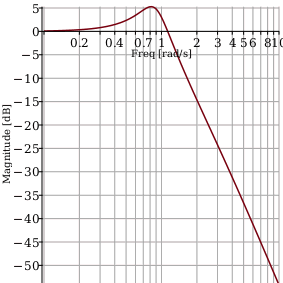DataFrames - Maple Help

 DataSeries and DataFrame

Maple 2016 adds two new data containers: DataSeries and DataFrame. These labeled tabular data structures are ideal for storage of many different kinds of data:

 • Tabular data with heterogeneous columns data types
 • Ordered or unordered data, including time series or sequential data
 • Any kind of statistical or observational data; labels are not essential for the data frame

DataSeries and DataFrames are built for easy manipulation and analysis of data. There are many commands in the Maple language that can be applied to these structures, including most Statistics commands. Many commands are also available from the right-click context menu. DataSeries and DataFrames also contain many commands, such as:

 • Account for missing values using the FillMissing and DropMissing commands
 • Find and remove duplicate entries using the AreDuplicate and AreUnique commands
 • DataFrames are mutable; add rows or columns with Append
 • Compute Aggregate statistics based on values in a column
 • convert DataSeries and DataFrames to various other data storage types and change the datatype in place for DataSeries
 • Subset and index into data using a natural labeled index or various Boolean queriesDataSeries

 • A DataSeries is a one-dimensional sequence of data with a label for each data point. For example, you can keep track of nutritional energy values (in $⟦\mathrm{kJ}⟧$ per 100 $⟦g⟧$) of certain types of berries, as follows:
 > energy := DataSeries(<220, 288, 136>, labels = [Raspberry, Grape, Strawberry]);• This allows you to access the energy values by position (number) or label (name).
 > energy;
 ${288}$ (1)
 > energy[Strawberry];
 ${136}$ (2)
 • You can determine which values satisfy some criteria by using elementwise operators. The result is a DataSeries of true/false values.
 > energy >~ 200;• You can use this DataSeries to filter the entries in the original DataSeries.
 > energy[energy >~ 200];DataFrame

 • A DataFrame is a two-dimensional rectangular table of data with a label for each column and for each row. For example, you can keep track of various properties of certain types of berries as follows:
 > genus := <"Rubus", "Vitis", "Fragaria">:
 > carbohydrates := <11.94, 18.1, 7.68>:
 > total_tons := < 543421, 58500118, 4594539 >:
 > top_producer := < Russia, China, USA >:
 > berry_data := DataFrame([genus, energy, carbohydrates, total_tons, top_producer], columns = [Genus, Energy, Carbohydrates, Total tons, Top producer], rows = Labels(energy));Note that in the above example, the data stored in the DataFrame is heterogeneous; each DataSeries has a different data type: Float, Integer, string, and name.

 • You can access columns by indexing the berry DataFrame with a number, for the position, or a name. Each column is a DataSeries.
 > berry_data;> berry_data[Carbohydrates];• Because columns are DataSeries, you can test properties like for DataSeries.
 > berry_data[Energy] >~ 200;• You can also filter rows. This returns a new DataFrame with a subset of the data.
 > berry_data[berry_data[Energy] >~ 200];• By using the with command, you can simplify the syntax a little: the column names then represent the corresponding column directly, without the use of indexing.
 > with(berry_data);
 $\left[{\mathrm{Genus}}{,}{\mathrm{Energy}}{,}{\mathrm{Carbohydrates}}{,}{\mathrm{Total tons}}{,}{\mathrm{Top producer}}\right]$ (3)
 > Carbohydrates;> berry_data[Energy >~ 200];More Details and Examples

 • You can find more details and examples on the following help pages:
 – The DataFrame Guide gives a detailed list of many of the more popular operations supported by DataFrames.
 – The DataSeries indexing and DataFrame indexing help pages describe the many other ways to use indexing with these two data structures in some detail.
 – The DataSeries and DataFrame help pages give an overview of the data structures.
 – The Dataframe Statistics page gives an overview of many common statistical operations on data frames.